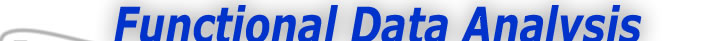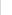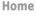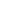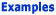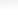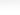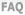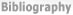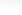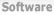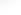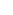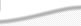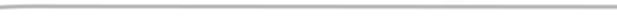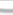BasisFunctions The Basics of Basis Functions The Characteristics of Spline Functions -What is a Spline Function -How do I choose the order m of a spline? Goods Growth Weather
 The Characteristics of Spline Functions Previous - 1 - 2 - Next Expertise: Intermediate How do I choose the order m of a spline? The most common type of spline is the cubic spline in which each polynomial is a cubic, or of order 4. Because the segments join with matching derivatives up to order 2, they appear to the eye to be beautifully smooth. This is because the second derivative measure the curvature of a curve, and the curvatures match at the breakpoints, so that the curvature appears to change smoothly. However, the order m that we use depends on how many derivatives we will need to compute from the spline function. A cubic spline is smooth in itself, but its first derivative will appear to change with noticeable abruptness at the breakpoints, and its second derivative will be a polygonal line. What this means is that if we want a smooth second derivative, we had better use a spline of order at least six, so that its second derivative will be at least smooth as a cubic spline. How do I choose the breakpoints ξl? The more breakpoints, the more flexible the spline. Moreover, this principle applies locally; if we need a lot of flexibility in a particular region of t, we use more breakpoints in this region. And, of course, less where we don't need much curvature. However, it is pointless to have breakpoints without data. There should in most situations be at least one observed value ti within each subinterval. If if one suspects that there is a sharp feature in a particular region, only one or two data values in its vicinity will pretty much eliminate any hope of adequately describing it, and the fitted curve may as well be smooth. The problem of deciding exactly where to position breakpoints is often finessed in one of two ways. First, users often just make them equally spaced, but of course paying attention to the requirement of having at least one observation in every subinterval. The second strategy is the place a breakpoint at every fixed number of observed values of t, sometimes called quantile placement. This has the advantage of ensuring that there is a reasonable amount of data associated with each subinterval. Figure 2 shows a typical situation in which some discrete noisy data are fit by a cubic spline function. The breakpoints are as shown, and they are spaced closer together in the middle where there is obvious more curvature needed than at the extremes.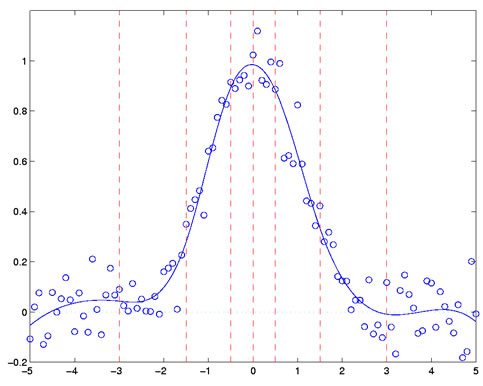Figure 2: The fit to some discrete noisy data, indicated by circles, achieved by a cubic spline function defined by the breakpoint values indicated by the vertical red dashed lines. What about spline basis functions? This discussion only gives a vague idea of how to construct spline functions, and does not address the issue of how a basis function system can be set up, linear combinations of which define any spline of a specified order and number of breakpoints. What we do have, though, is an explanation of why splines are so handy for curve fitting. They can be evaluated just as rapidly as polynomials because, at any point t, that is exactly what they are. Their derivatives, unlike those of polynomials, can be made as flexible as we wish by increasing the order of the spline. We do need to look at spline basis functions, though, to see why the coefficients ak can be computed rapidly no matter how many breakpoints are used. Go to the next web site in this series to look at spline basis functions. However, if you simply need to work with spline functions and are content to leave the properties of the spline basis functions to the experts, you probably already know enough. Previous - 1 - 2 - Next